• B样条曲线 1、B样条曲线的由来 在B样条曲线诞生之前，先有的是贝塞尔曲线（Bezier），由于贝塞尔曲线的一部分不足，引出了B样条曲线。 1.1 贝塞尔曲线的由来 给定n+1{n+1}n+1个数据点，p0p_0p0​~pnp_npn​，能够...
B样条曲线
1、B样条曲线的由来
在B样条曲线诞生之前，先有的是贝塞尔曲线（Bezier），由于贝塞尔曲线的一部分不足，引出了B样条曲线。
1.1 贝塞尔曲线的由来
给定${n+1}$个数据点，$p_0$~$p_n$，能够生成一条曲线，使得该曲线与这些点描述的形状相符。
1.2 贝塞尔曲线的定义
$\ {p_t}=\sum_{i=0}^n p_i B_{i,n}(t)\qquad (1)$
Bezier曲线的基函数可以简化成伯恩斯坦基函数$B_{i,n}(t)$，其中
$\ B_{i,n}(t)=\frac{n!}{i!{(n-i)}!}t^i(1-t)^{n-i} , t\in[0,1]\qquad (2)$
贝塞尔的参数B是二项式$(t+(1-t))^n = (1)^n$的展开公式。后面的很多的贝塞尔曲线的性质都可以用这个来解释。
二项式定理：
$(x+y)^n = \sum_{i=0}^nC_n^ix^{n-i}y^i$
根据此定理，可以将$x+y$的任意次幂展开成和的形式
贝塞尔曲线可以通过递归的方式来绘制，也更便于理解。
贝塞尔曲线的导数还是贝塞尔曲线。
1.3 贝塞尔曲线的例子
（1）一次Bezier曲线
当$n=1$时，有两个控制点$p_0$和$p_1$，Bezier多项式是一次多项式：
$\ {p_t}=\sum_{i=0}^1 p_i B_{i,1}(t)=p_0 B_{0,1}(t)+p_1 B_{1,1}(t)=(1-t)p_0+tp_1\qquad (3)$
即为连接起点$p_0$和终点$p_1$的直线段。
（2）二次Bezier曲线
当$n=2$时，有3个控制点p0、p1和p2，Bezier多项式是二次多项式：
$\ {p_t}=\sum_{i=0}^2 p_i B_{i,2}(t)=p_0 B_{0,2}(t)+p_1 B_{1,2}(t)+p_2 B_{2,2}(t)=(1-t)^2p_0+2t(1-t)p_1+t^2p_2\qquad (4)$
2、B样条曲线的定义
B样条曲线的数学表达式为：
$\ {p_u}=\sum_{i=0}^n p_i B_{i,k}(u), u\in[u_{k-1},u_{n+1}]\qquad (5)$
$B_{i,k}(u)$称为$k$阶$(k-1)$次B样条基函数，k是刻画次数的。其中k可以是2到控制点个数$n+1$之间的任意整数。
对Bezier曲线来说，阶数和次数是一样的；但对B样条，阶数是次数加1。
B样条基函数是一个称为节点矢量的非递减的参数u的序列所决定的k阶分段多项式，这个序列称为节点向量。
与贝塞尔曲线类似，B样条曲线也可以用递归的方式定义。
de Boor-Cox递推定义
它的原理是，只要是$k$阶$k-1$（次）的B样条基函数，构造一种递推的公式，由0次构造1次，1次构造2次，2次构造3次，依此类推。
$B_{i,1}(u)=\begin{cases} 1 , u\in(u_{i},u_{i+1})\\ 0, 其它 \end{cases}\qquad (6)$
$\ B_{i,k}(u)=\frac{u-u_i}{u_{i+k-1}-u_i}B_{i,k-1}(u)+\frac{u_{i+k}-u}{u_{i+k}-u_{i-1}}B_{i+1,k-1}(u)\qquad (7)$
并约定
$\frac{0}{0}=0$
该递推公式表明：若确定第$i$个$k$阶B样条$B_{i,k}(u)$，需要用到$u_i,……u_{i+k}$,共$k+1$个节点，称区间$[u_i,u_{i+k}]$为$B_{i,k}(u)$的支撑区间。
支撑区间指的是使$B_{i,k}(u)$不为0的区间。
3、B样条基函数定义区间及节点向量
合法的定义区间必须满足在该区间有足够多的基函数不为0，利用B样条基函数递推定义，据此推出B样条基函数的定义区间为$[u_{k−1​},u_{n+1​}]$。同时阶数$k$加控制点$n+1$为节点向量的个数。
4、B样条曲线是多段贝塞尔曲线拼接的理解
假设控制点的个数为$n+1$，阶数为$k$，根据递推定义则有：
$p_0 B_{0,4}(u)$，涉及u_0到u_4个节点
$p_1 B_{1,4}(u)$，涉及u_1到u_5个节点
$p_2 B_{2,4}(u)$，涉及u_2到u_6个节点
$p_3 B_{3,4}(u)$，涉及u_3到u_7个节点
$p_4 B_{4,4}(u)$，涉及u_4到u_8个节点
所以B样条曲线被分成俩段：$u_3$$u_4$，$u_4$$u_5$。
刚好$p_0$、$p_1$、$p_2$、$p_3$对应的基函数是在$u_3$$u_4$区间里有定义，$p_1$、$p_2$、$p_3$、$p_4$对应的基函数是在$u_4$$u_5$区间里有定义。
因为B样条曲线是3次的，这里单个节点区间又对应了4个有效的控制点，则在单个节点区间里的B样条曲线可以用贝塞尔曲线代替。因为$4$个控制点会有一条$3$阶的贝塞尔曲线，目的是找一条逼近控制点的曲线，这样就找到了。又因为相邻俩段之间有三个控制点是一样的，这样就会保证相邻俩段贝塞尔曲线的拼接效果非常好。
即每一个有效定义的节点区间内都可以看作一个贝塞尔曲线。
可以用贝塞尔曲线B样条曲线代替单个节点向量区间对应的曲线是因为对应的控制点一样，同时贝塞尔曲线与控制点的逼近效果很好。
参考：
https://blog.csdn.net/qq_40597317/article/details/81155571
https://blog.csdn.net/weixin_42513339/article/details/83118099
https://blog.csdn.net/weixin_45971567/article/details/105629924


展开全文•研究论文
• B样条曲线逼近代码，是matlab代码，B样条曲线逼近代码，是matlab代码，B样条曲线逼近代码，是matlab代码，B样条曲线逼近代码，是matlab代码，
• ## 三次B样条曲线拟合算法

万次阅读 多人点赞 2017-01-17 22:10:28
三次B样条曲线方程B样条曲线分为近似拟合和插值拟合，所谓近似拟合就是不过特征点，而插值拟合就是通过特征点，但是插值拟合需要经过反算得到控制点再拟合出过特征点的B样条曲线方程。这里会一次介绍两种拟合算法。...
1 三次B样条曲线方程
B样条曲线分为近似拟合和插值拟合，所谓近似拟合就是不过特征点，而插值拟合就是通过特征点，但是插值拟合需要经过反算得到控制点再拟合出过特征点的B样条曲线方程。这里会一次介绍两种拟合算法。首先介绍B样条的曲线方程。B样条曲线的总方程为：P(t)=∑i=0nPiFi,k(t)P(t)=\sum_{i=0}^{n} P_{i}F_{i,k}(t)    (1)
其中PiP_i是控制曲线的特征点，Fi,k(u)F_{i,k}(u)则是K阶B样条基函数。1.1 三次B样条曲线方程中基函数为：Fi,k(t)=1k!∑m=0k−i(−1)m(mk+1)(t+k−m−j)kF_{i,k}(t)=\frac{1}{k!}\sum_{m=0}^{k-i}(-1)^{m}\binom{m}{k+1}(t+k-m-j)^k  (2)
其中(mk+1)\binom{m}{k+1}表示阶乘，化成看的明白的式子就是：
（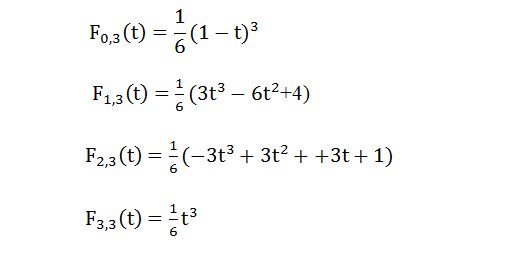将图片上的基函数代入到方程（1）中，就是：P(t)=P0∗F0,3(t)+P1∗F1,3(t)+P2∗F2,3(t)+P3∗F3,3(t)P(t)= P_0*F_{0,3}(t)+P_1*F_{1,3}(t)+P_2*F_{2,3}(t)+P_3*F_{3,3}(t)  (3)
方程（3）就是三次B样条曲线方程。

2019-04-18 更新

有小伙伴提到上式(2)的j是什么意思，其实j就是控制点的索引值。这里我把书上的公式摘抄下来，可能看起来更为清晰。
参考书籍：《计算机图形学 第3版》何援军 第13章三次B样条曲线计算F0,3(t)=13!∑j=03(−1)jC4j)(t+3−0−j)3F_{0,3}(t)=\frac{1}{3!}\sum_{j=0}^{3}(-1)^{j}C^{j}_{4})(t+3-0-j)^3
=16[(−1)0C40(t+3)3+(−1)1C41(t+2)3+(−1)2C42(t+1)3+(−1)3C43t3]=\frac{1}{6}[(-1)^{0}C^{0}_{4}(t+3)^{3}+(-1)^{1}C^{1}_{4}(t+2)^{3}+(-1)^{2} C^{2}_{4}(t+1)^{3}+(-1)^{3}C^{3}_{4}t^{3}]
=16(−t3+3t2−3t+1)=16(1−t)3=\frac{1}{6}(-t^{3}+3t^{2}-3t+1)=\frac{1}{6}(1-t)^{3}
F1,3(t)=13!∑j=02(−1)jC4j)(t+3−1−j)3F_{1,3}(t)=\frac{1}{3!}\sum_{j=0}^{2}(-1)^{j}C^{j}_{4})(t+3-1-j)^3
=16[(−1)0C40(t+2)3+(−1)1C41(t+1)3+(−1)2C42t3]=\frac{1}{6}[(-1)^{0}C^{0}_{4}(t+2)^{3}+(-1)^{1}C^{1}_{4}(t+1)^{3}+(-1)^{2} C^{2}_{4}t^{3}]
=16(3t3−6t2+4)=\frac{1}{6}(3t^{3}-6t^{2}+4)
F2,3(t)=13!∑j=01(−1)jC4j)(t+3−2−j)3F_{2,3}(t)=\frac{1}{3!}\sum_{j=0}^{1}(-1)^{j}C^{j}_{4})(t+3-2-j)^3
=16[(−1)0C40(t+1)3+(−1)1C41t3=\frac{1}{6}[(-1)^{0}C^{0}_{4}(t+1)^{3}+(-1)^{1}C^{1}_{4}t^{3}
=16(−3t3+3t2+3t+1)=\frac{1}{6}(-3t^{3}+3t^{2}+3t+1)
F3,3(t)=13!∑j=00(−1)jC4j)(t+3−3−j)3F_{3,3}(t)=\frac{1}{3!}\sum_{j=0}^{0}(-1)^{j}C^{j}_{4})(t+3-3-j)^3
=16[(−1)0C40t3=\frac{1}{6}[(-1)^{0}C^{0}_{4}t^{3}
=16t3=\frac{1}{6}t^{3}
2 三次B样条曲线近似拟合
近似拟合很简单。不需要求控制点，求得Fi,k(t)F_{i,k}(t)，由上述方程（3），代入P0,P1,P2,P3P_0,P_1,P_2,P_3就可以得到由这四个点近似拟合的一段三次B样条曲线，起始点在P0P_0，终点在P1P_1，对于闭合轮廓，最后一段可以取前两点做辅助，拟合实验结果我最后一块给出。这种近似拟合曲线光滑，但是最大不足就是不过特征点，也就是不过PiP_i，需要过点需要反求控制点再拟合。
3 三次B样条插值拟合
插值拟合较为复杂。其实也不算是很复杂，找资料过程和理解过程是一个复杂的过程。不过有了前面大神做工作，我们只是借用别人的成果写代码就好了。我给大家看一篇论文，大家可以百度或者去知网搜索，闭合 B 样条曲线控制点的快速求解算法及应用。文章讲解了反求控制点的具体步骤，写的非常详细，基本上贴近代码的那种。大家可以根据这篇论文反求控制点，拟合出来的三次B样条曲线是经过PiP_i的。代码就不放了，很多，可以根据我给的那篇论文直接编写相应代码，有问题可以私信我，知无不言。
##4 拟合结果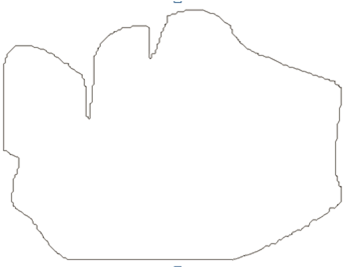原轮廓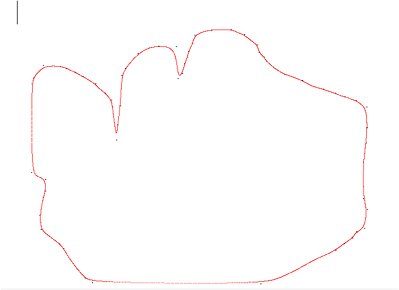近似拟合轮廓。可以看到没过黑色特征点，只是近似拟合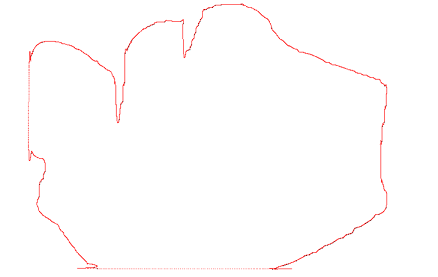插值拟。可以看到曲线经过黑色特征点，不过有一些不足之处。
##5 总结
三次B样条曲线拟合轮廓效果还是可以，较之Beizer（可以参考我博客三次Beizer曲线拟合算法），B样条将一些细节描述的很好，很多细节之处都贴近原轮廓，但是有一些不足之处，可以看到对直线拟合效果不是很好。两篇博客都是关于闭合轮廓的拟合，对于非闭合或者只是一段曲线拟合，还有一种曲线是很好的，《数值分析》提到过，叫三次样条插值拟合，拟合效果很好，我做过拟合一元三次方程曲线，拟合效果跟原曲线非常贴近，不过过程中需要用到追赶法，而追赶法需要满足一个条件，对于闭合曲线三次样条插值是不满足这个条件的，所以我没去深研究，大家可以去试一试。谢谢大家！
-------------------------------------------------2018-10-30--------------------------------------------
真的很感谢大家的支持，这一年都比较忙，找实习and找工作，而且这个东西是研一上学期搞的，现在看都毫无印象，对自己说一句：牛逼。我把代码放在网盘了（能运行，但现在没效果，大家可以检查下，之前是OK的），本来想上传到CSDN，好像要C币，而且要审核，太墨迹了。
链接: https://pan.baidu.com/s/1mSQMmvL71gwEAqgiT6O9Gg 提取码: xv5f


展开全文openCV
• 利用VB实现B样条曲线，实现步骤：首先判断u所有范围，然后计算该范围内所有非零的基函数Nip，最后计算出B样条曲线上点的坐标
• B样条曲线拟合。实现简单高效，项目中实际使用的代码。
• 1、掌握Bezier曲线的绘制； 2、掌握B样条曲线的绘制。 通过菜单完成两种曲线的绘制:
•研究论文
• ## B样条曲线拟合原理

万次阅读 多人点赞 2017-01-13 18:51:43
B样条曲线是在Bezier 曲线基础上发展起来的一类曲线，它克服了Bezier 曲线整体控制性所带来的不便，最常用的是二次和三次B样条曲线。 2.二次B样条 2.1 参数方程 已知三个平面离散点P0、P1、P2，由这三点可以定义...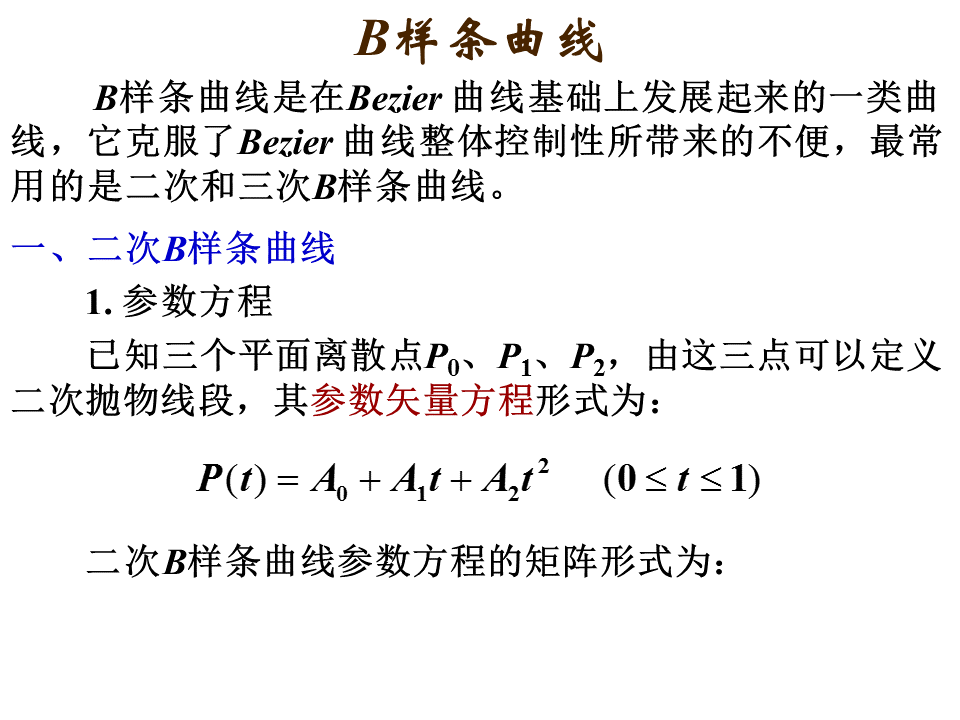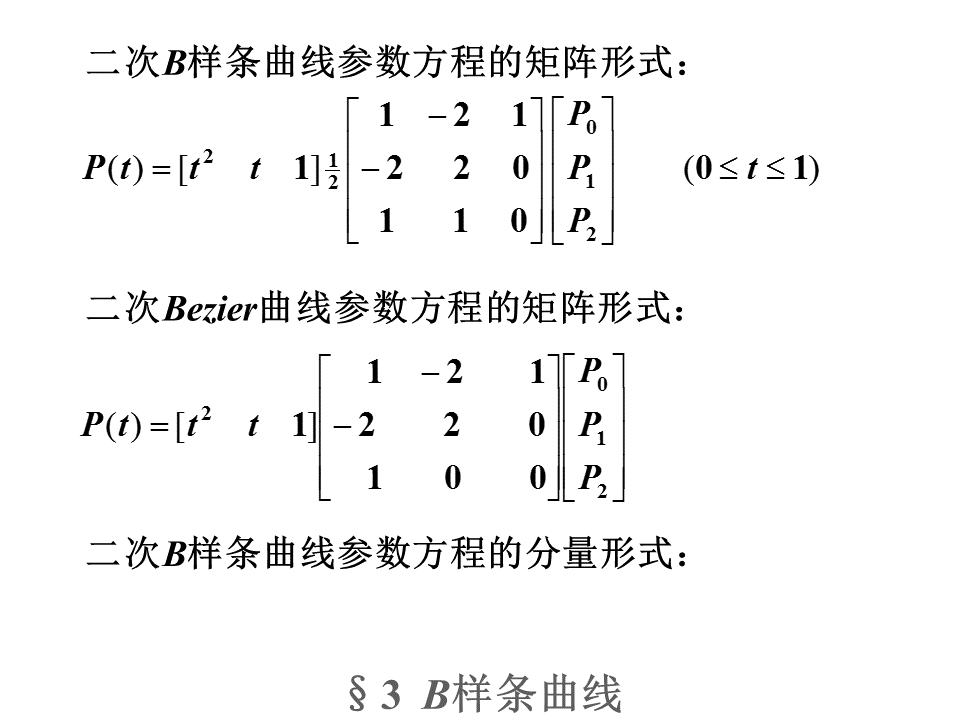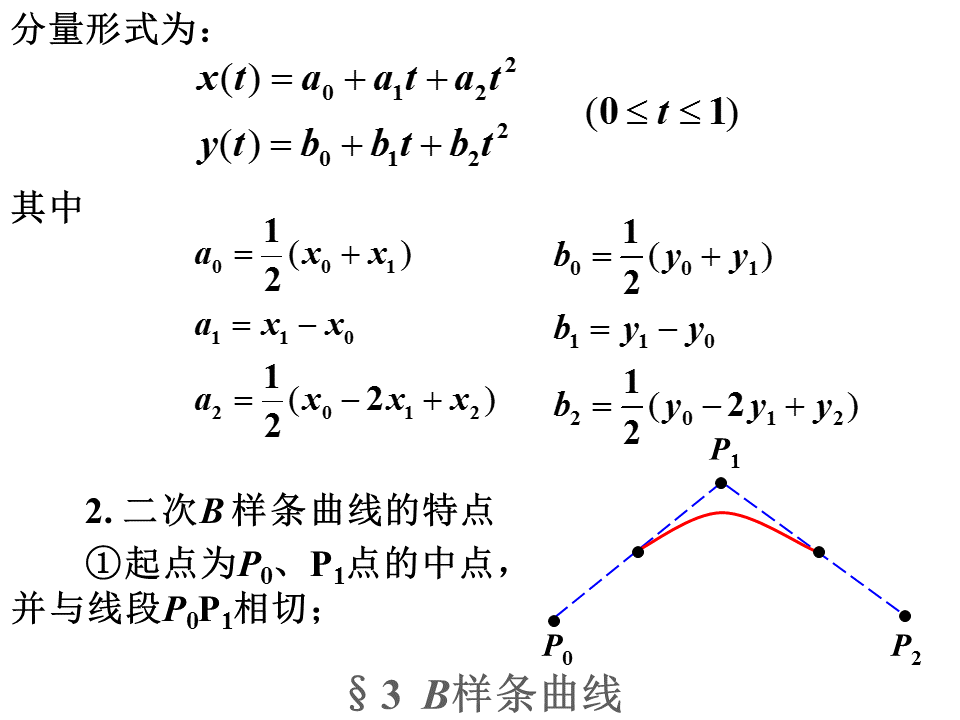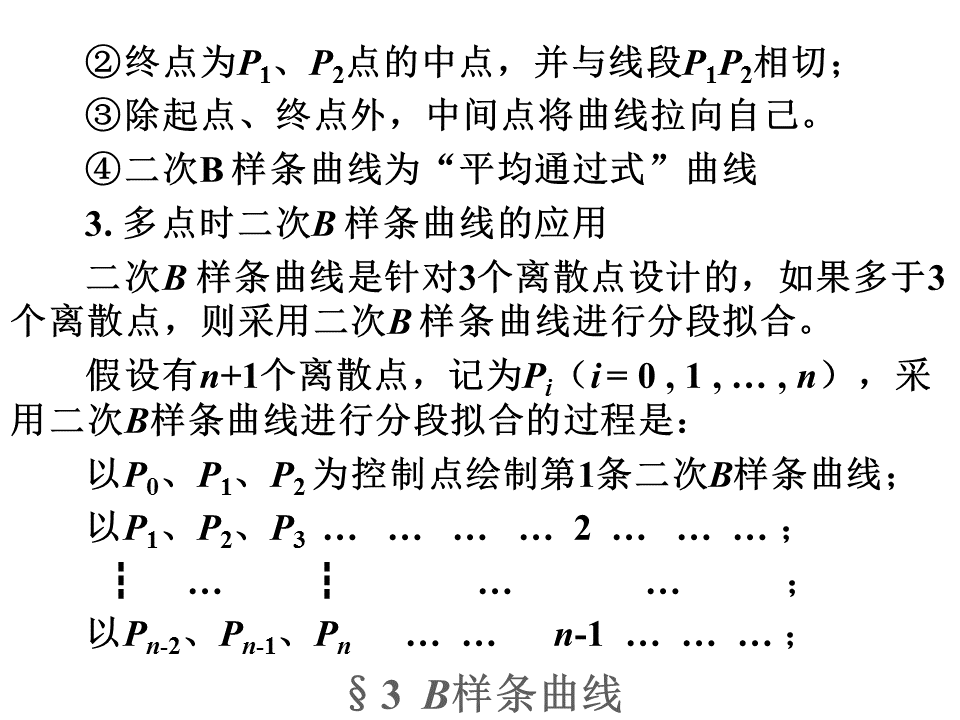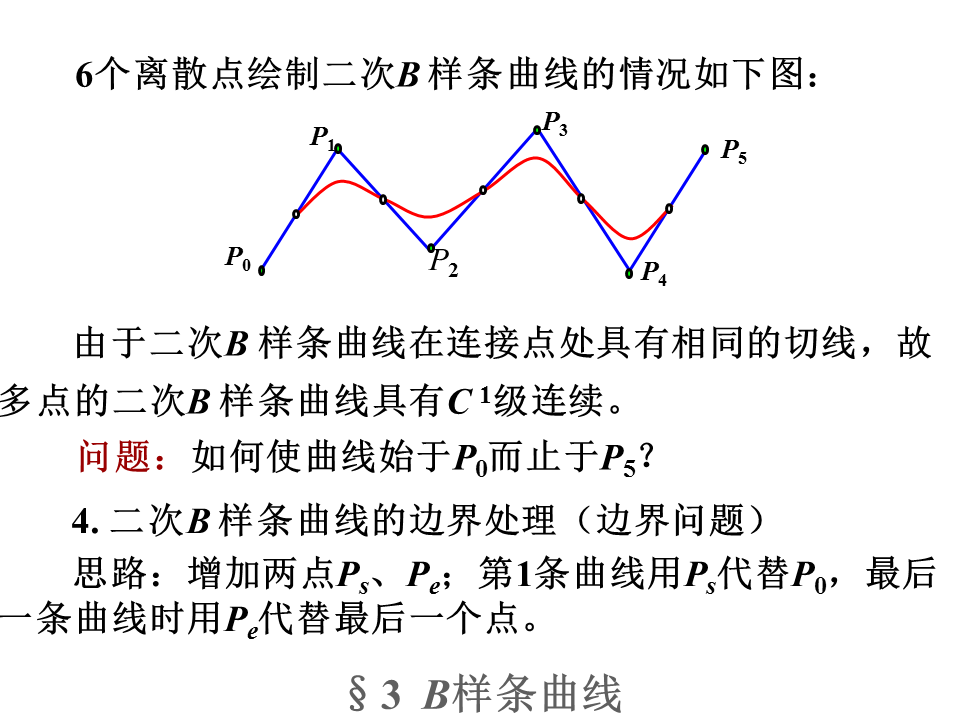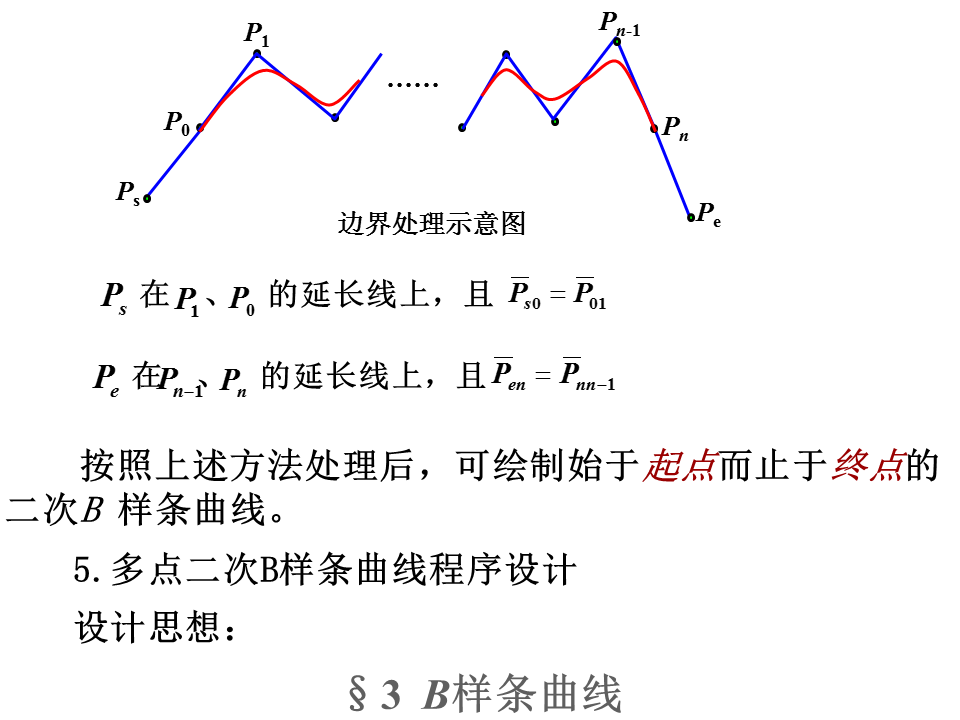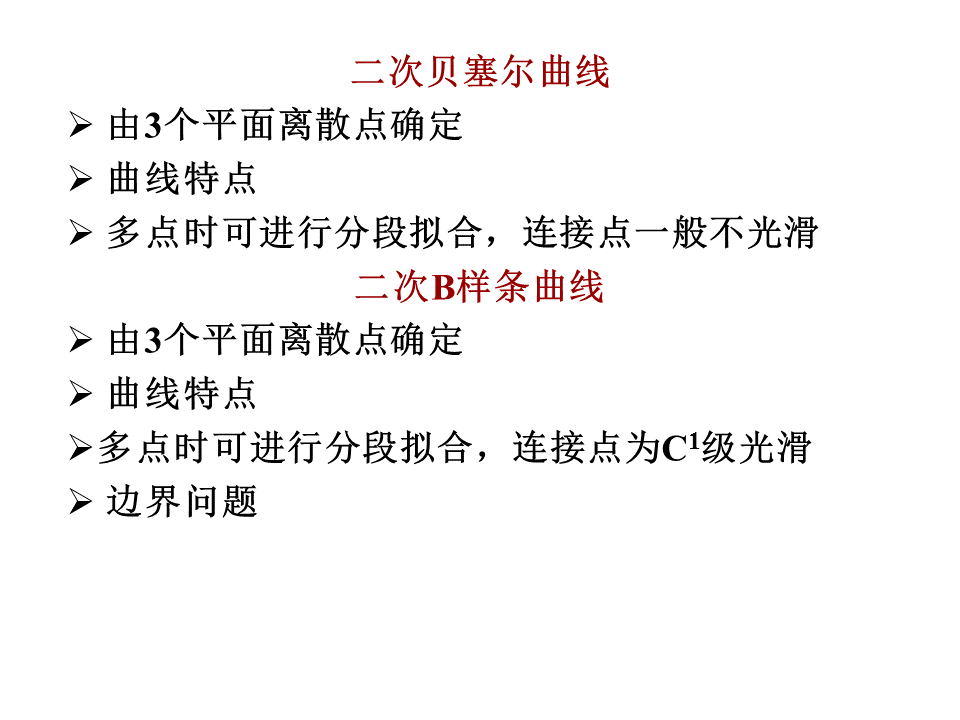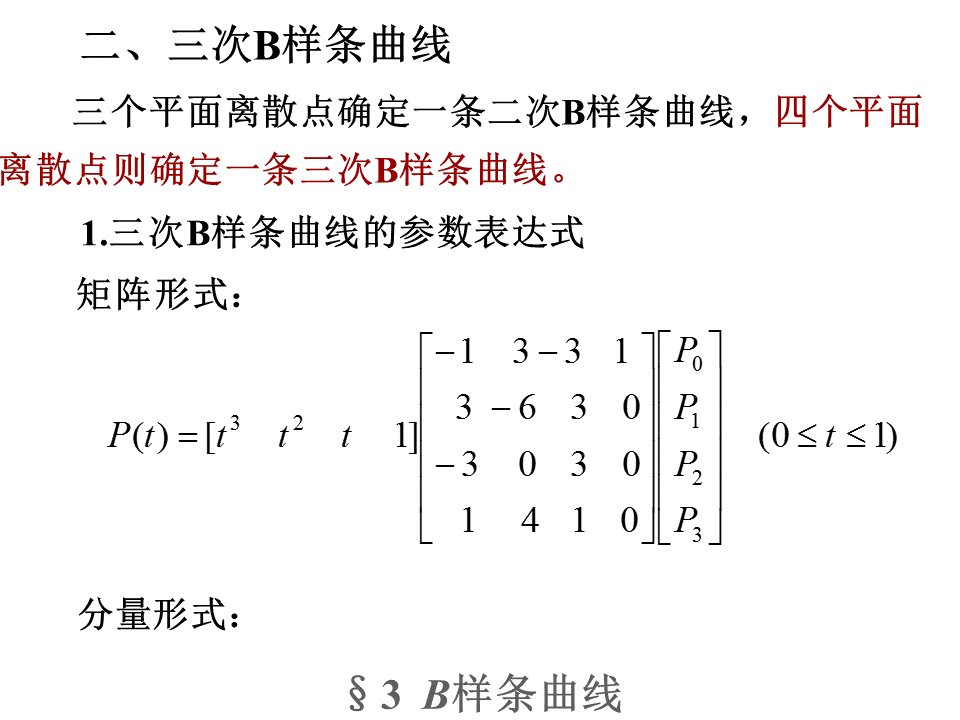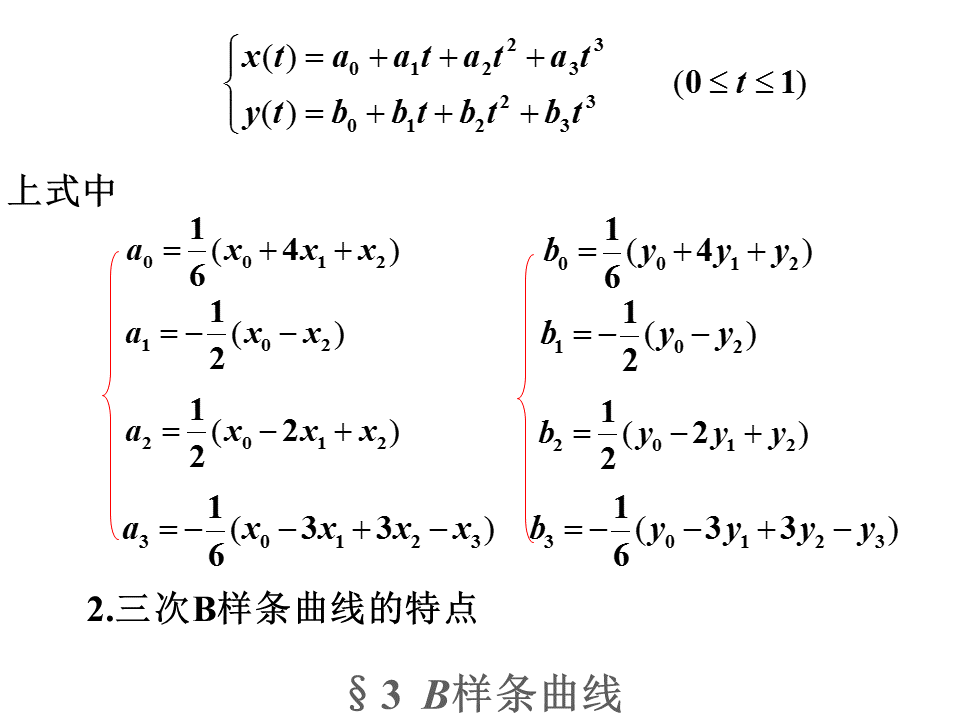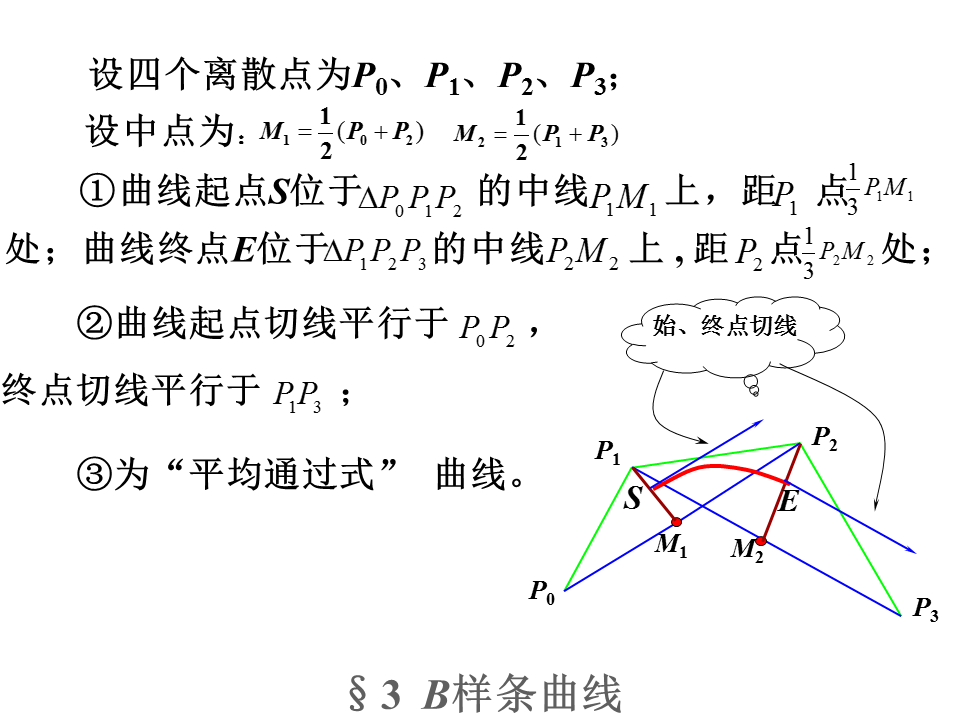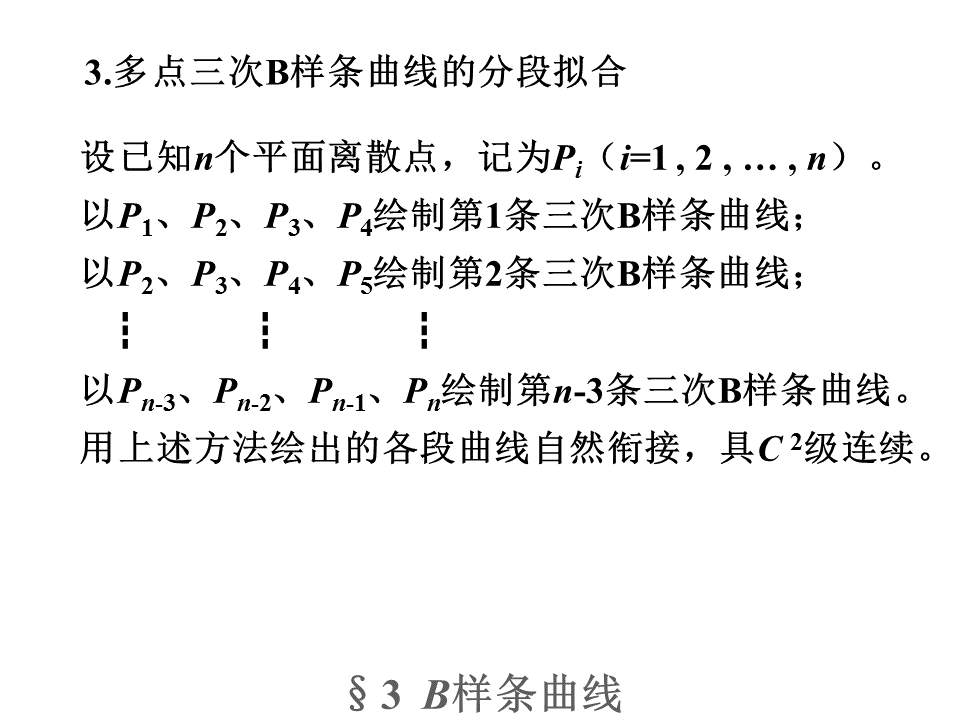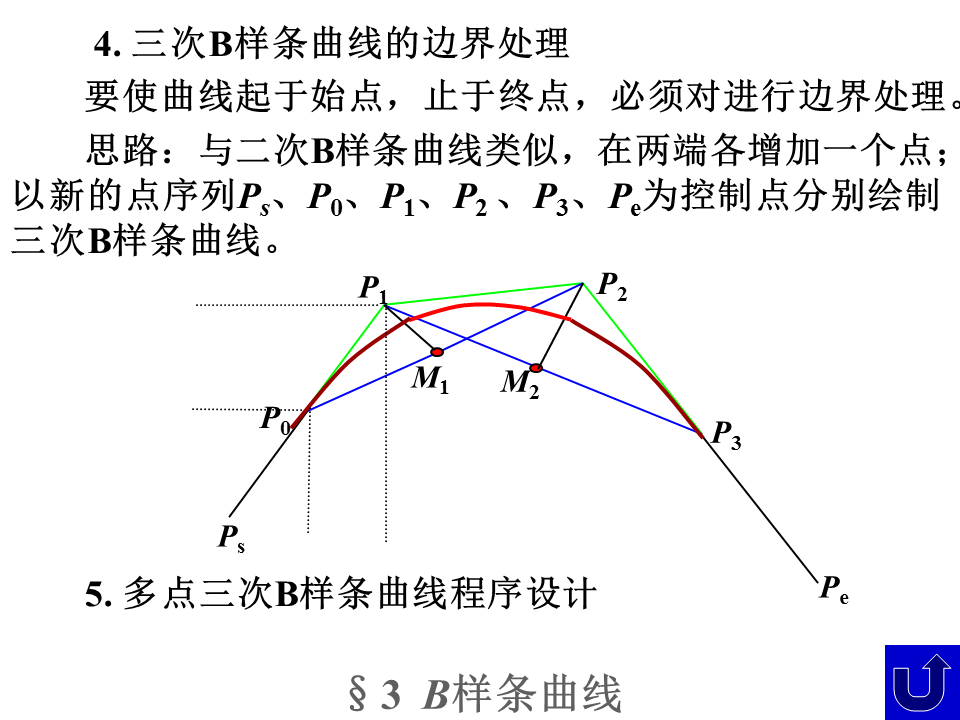展开全文• ## B样条曲线（B-spline Curves）

万次阅读 多人点赞 2018-07-27 16:34:38
关键字：NURBS，基函数，控制点，节点，

另一个讲的很好的 https://www.cnblogs.com/icmzn/p/5100761.html

看了网上很多相关资料才得以下笔，资料太多，这里就不一一列举了，感谢各位大佬的资料

本博客顺序不太好，看前面的东西可能需要提前看后面的东西。正在努力修炼，敬请谅解

写了个B样条曲线计算的完成程序，包括绘图，https://download.csdn.net/download/qq_40597317/10646881，BaseFunction部分参考了https://www.cnblogs.com/nobodyzhou/p/5451528.html 侵删 重点就是由于规定了0/0=0，所以最需要注意的地方，原作者的思路是如果除数为0，就让除数为1，然后继续运算。这个思路真的很棒，如果被除数为0的话，那么结果就是0，否则的话结果就是被除数了。显然也没有人要求到这点。运行图像结果如下：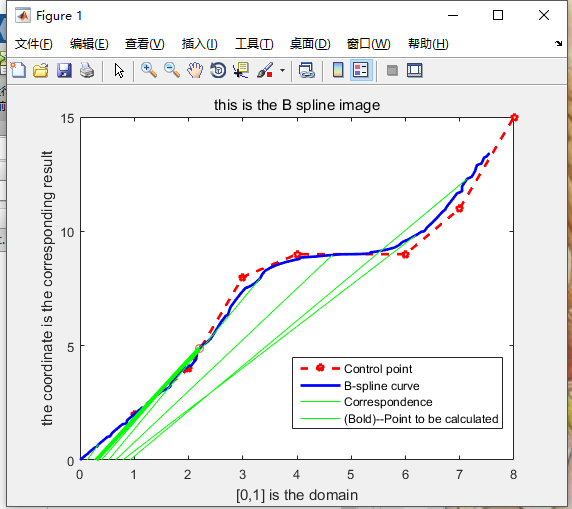一、定义

1.1 概述

是B-样条基曲线（给定区间上的所有样条函数组成一个线性空间。这个线性空间的基函数就叫做B样条基函数）的线性组合。

B-样条是贝兹曲线的一种一般化，B样条不能表示一些基本的曲线，比如圆，所以引入了NURBS,可以进一步推广为非均匀有理B-样条(NURBS)。三者关系可以表示为：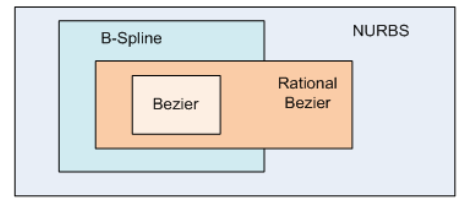细分定义域

直接细分（Subdividing）曲线是很困难的。因此，我们细分曲线的定义域。因此，如果曲线的定义域是[0,1]，这个闭区间被称为节点（knots）的点细分而成。设这些节点是 0 <= u0 <= u1 <= ... <= um <= 1。那么点C(ui)的曲线细分如下图所示，因此，修改[0,1]的细分会改变曲线的形状。

如下图，这里有5段曲线组成了整个曲线。下面的直线定义域为[0,1]，就是[0,1]被分成五段

可以看出，这里定义域被节点细分，节点分别是0, 0.2, 0.4, 0.6, 0.8,1，6个节点正好可以把定义域（即下面的黑色线段）分成5段。

可以看出，6个节点分别对应于曲线上的一个点,可以用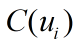代表对应的曲线上的点，被称为节点点(knot point)，节点点把B-样条曲线划分成5个曲线段，每个都定义在一个节点区间上。这些曲线段都是 p 次的贝塞尔曲线。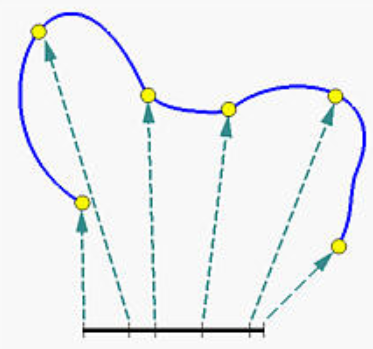节点(knots)

有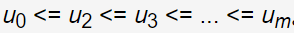那么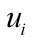被称为节点，显然上述的m=5

设U是m+1个非递减数的集合，那么有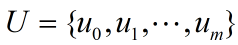，集合U称为节点向量(knot vector)。

如果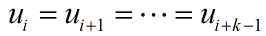，那么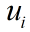是一个重复度(multiplicity)为k的多重节点,k>1,否则如果一个节点只出现一次，那么这就 是一个简单节点。如果节点等间距，节点向量或节点序列称为均匀的；否则它是非均匀的。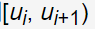是第i节点个区间,i=0,1,...,m

所有的B样条基函数都被假设在定义域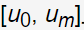上，通常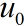为0，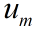为1

1.2 组成

总之，为了设计一个B-样条曲线，我们需要一系列的控制点（就是下图折线的连接点，一共8个），一系列的节点和一系列的系数，每个系数对应一个控制点，所以所有曲线段连接在一起满足某个连续 条件。系数的计算可能是最复杂的步骤因为它们必须保证某个连续条件。

将多个贝塞尔曲线连接就可以得到B样条。

如下图所示，这里有8个控制点，依次用线段连接，B样条曲线由一系列5条3次的贝塞尔曲线连接形成。

一般次数越低（即p越小），那么B样条曲线就更容易逼近他的控制折线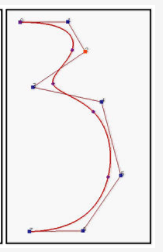二、特点

2.1 一般不经过控制点

B样条由一系列控制点决定，但是B样条不会经过其控制点。

2.2 分段

以上所有的分段都为Bezier曲线，对于分段Bezier曲线，不同的曲线段相互独立，移动控制点只会影响其所在的Bezier曲线段，而其他的Bezier曲线段都不会改变，甚至所有关于Bezier曲线的算法可以同样地适用于分段Bezier曲线。

核心思想就是：用分段低阶多项式通过连续的连接来代替高阶多项式

2.3 与Bezier对比

Bezier曲线/曲面不支持局部的修改和编辑；
Bezier曲线/曲面拼接时，满足几何连续条件是十分困难的。

三、数学表达

3.1 一般表达

有n+1个控制点Pi(i=0,1,...,n)和一个节点向量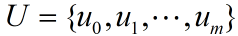，依次连接这些控制点可以构成一个特征多边形，k+1阶（k次）B样条曲线的表达式为（2<=k<=n+1），必须满足m=n+k+1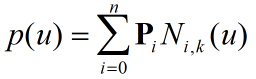其中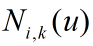是k次B样条基函数，也叫调和函数，或者 k次规范B样条基函数，下面为其递归公式（这个公式叫Cox-de Boor）的定义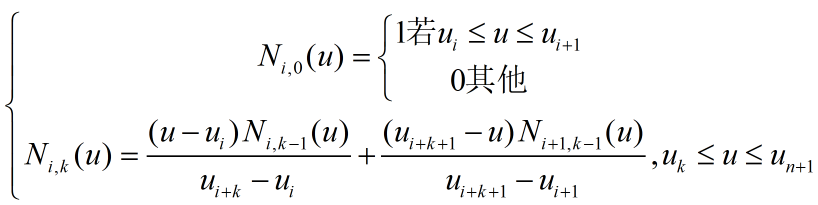显然，基函数由U定义，其中基函数满足微分方程：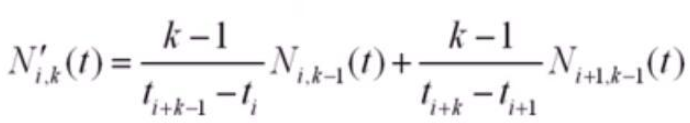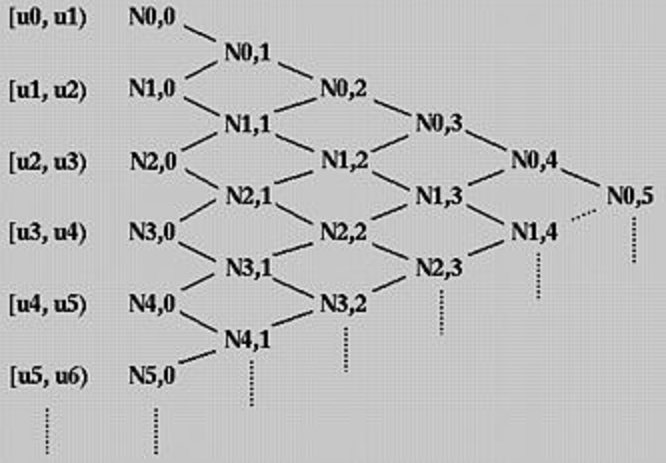上图是基函数的运算关系，从左向右，从上往下可以依次计算各个基函数

基函数：

基函数只在附近的一个子区间非0，这就是局部的概念。（不明白的）

基函数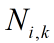表示基函数的次数(degree)为k，这是第i个k次B样条基函数,i=0,1,...

四、性质

4.1 基函数的性质

1. 局部支撑性

若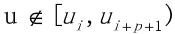，那么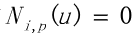2.

在任意给定节点区间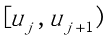,最多p+1个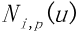非零，分别是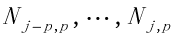3.非负性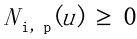4.规范性

任意给定节点区间,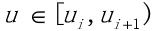时

可微性

在节点内部，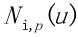无限次可微，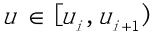时，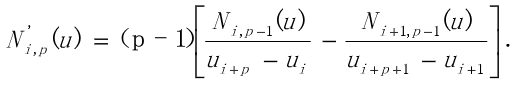4.2 凸包包含

样条曲线包含在控制折线（ployline）的凸包内。更特别地，如果u 在节点区间[ui,ui+1)里，那么C(u)在控制点Pi-p, Pi-p+1, ..., Pi的凸包里。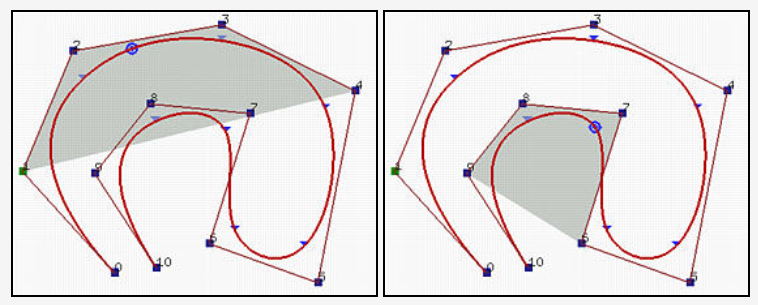4.3 Pi 只影响在区间[ui, ui+p+1)上的曲线 C(u)。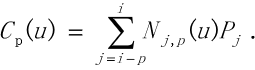五、分类（根据节点向量中节点的分布）

5.1 均匀B样条曲线

(这里的节点可以理解为控制点)

节点成等差数列均匀分布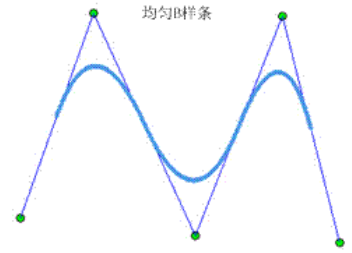5.2 准均匀B样条曲线

两端节点0,1重复度为次数k的基础上加1，即k+1，即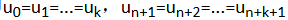，所有内部节点重复度为k+1.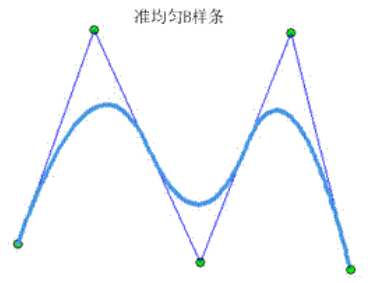显然，首尾两边是相切的

5.3 分段Bezier曲线

两端节点重复度为k+1，内部节点重复度为k，显然此时必须满足条件(m-1)%k==0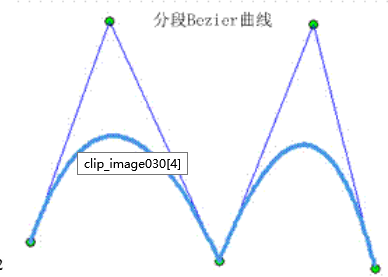5.4 非均匀B样条

任意选取的一个序列[u0,...,um]，只要在数学上成立即可，这是最一般的情况

六、计算B样条上的一点的值

7.1 修改曲线的形状

可以修改一个或多个控制参数：控制点的位置(n+1)，节点位置(m+1)，和曲线的次数p。

一般为开（open ）B-样条曲线，也就是产生的曲线不会与控制折线（polyline）的第一边（leg）和最后一边（leg）接触，如下图，对于开曲线，区间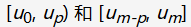不会有基函数的完全支持（“full support”）,就是指在这些区间上，只有一个非零基函数。所以有以下结论，对于开B样条曲线，定义域为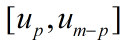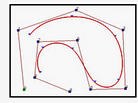强制第一个节点和最后一个节点的重复度为p+1，那么产生的曲线就会分别与第一个控制点和最后一个控制点的第一边和最后一边相切，如下图，这是clamped B-样条曲线。注意，Clamped B-样条曲线C(u)通过首尾两个控制点 P0 和Pn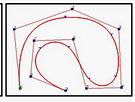通过重复某些节点和控制点，产生的曲线会是  闭（closed）曲线。会产生闭环，如下图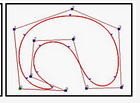如何产生闭曲线？

1.Wrapping控制点

(1)设计一个均匀 m+1 个节点的节点序列：u0 = 0, u1 = 1/m, u1 = 2/m, ..., um = 1。注意曲线的定义域是 [up, un-p].

(2)Wrap头p 个和最后p 个控制点。更准确地，设P0 = Pn-p+1, P1 = Pn-p+2, ..., Pp-2 = Pn-1 and Pp-1 = Pn.

构建的曲线在连接点处 C(up) = C(un-p)是Cp-1 连续的。.

1.Wrapping节点

1）增加一个新控制点 Pn+1 = P0.因此，控制点的数目是 n+2.

（2）找到一个合适的有 n+1节点的节点序列u0, u1, ..., un 。这些节点不必要是均匀的

（3）增加 p+2 个节点并 wrap 头 p+2个节点： un+1 = u0, un+2 = u1, ..., un+p = up-1, un+p+1 = up, un+p+2 = up+1 ，如下图所示。这样，我们有n+p+2 = (n+1) + p + 1 个节点

（4）定义在上述构建的 n+1个控制点和n+p+2 个节点上的 p 次开B-样条曲线C(u)是一个闭曲线，在连接点处C(u0) = C(un+1)有Cp-1 连续性。注意闭曲线的定义域是 [u0, un+1]

七、例子

7.1 简单节点

节点向量是U = { 0, 0.25, 0.5, 0.75, 1 }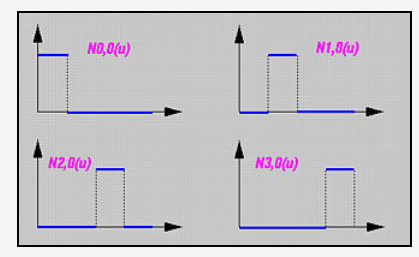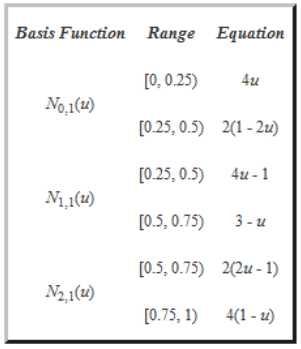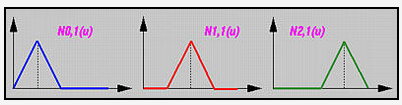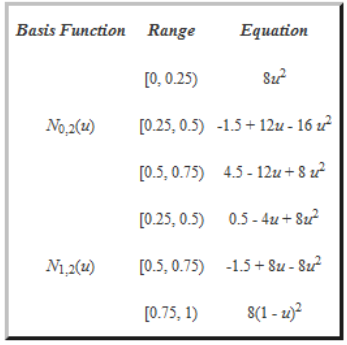7.2 带正重复度的节点

这个比较简单，略略略

（4）后面的主要是B样条曲线的一些性质，暂时用不到，后续更新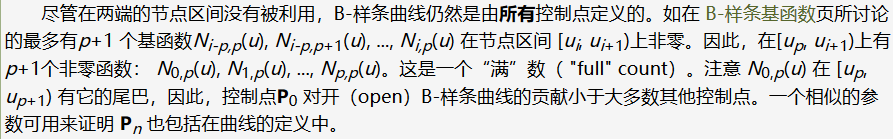展开全文• 计算机图形学课设，运用MFC编程技术，在vs平台，绘制Bzier曲线和B样条曲线
• ## B样条曲线曲面 Mfc

热门讨论 2012-02-26 11:08:27
本程序利用MFC实现了B样条曲线曲面的绘制：均匀B样条曲线 准均匀B样条曲线 分段Bezier曲线 非均匀B样条曲线 曲面
• 实现C++ opengl的glut库接口函数画贝塞尔曲线和均匀B样条曲线...